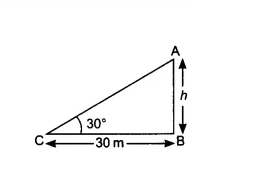# 4. The angle of elevation of the top of a tower from a point on the ground, which is 30 m away from the foot of the tower, is 30°. Find the height of the tower.

M manishLet the height of the tower AB is  and the angle of elevation from the ground at point C is
According to question,
In the right triangle ,

the value of  is  = 10(1.732) = 17.32 m
Thus the height of the tower is 17.32 m

Exams
Articles
Questions